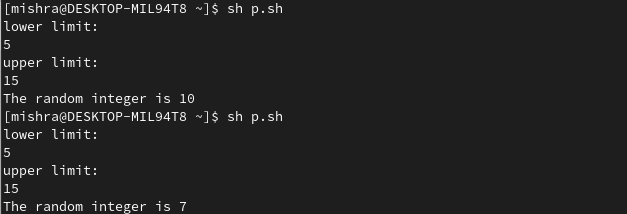# RANDOM Shell Variable in Linux with Examples

• Difficulty Level : Basic
• Last Updated : 10 Nov, 2020

RANDOM is a shell variable that is used to generate random integers in Linux. It is an internal bash command that returns a pseudo-random 16-bit integer in the range 0 – 32767. It returns a different integer at each invocation. To enhance the randomization process, the random variable can be seeded with an initial value which can be the time in epoch, the process ID of the current shell, etc.

Syntax to use time in epoch to initialize the generator:

```RANDOM=\$(date +%s)
```

Syntax to use process ID of the current shell to initialize the generator:

```RANDOM=\$\$ #PID of shell is stored in \$\$ variable
```

### Working with the RANDOM variable

Generating  random integer

Random integer can be generated by directly reading from the random variable by using the echo command. It will display a random integer in range 0 to 32767.

```echo \$RANDOM
```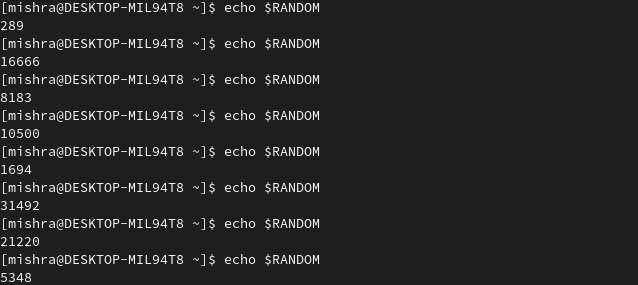Generating ‘n’ random integers

You may use loops to generate more than one random number using the RANDOM shell variable. The following code will generate 5 random values in the range from 0 to 32767.

```RANDOM=\$\$
for i in `seq 5`
do
echo \$RANDOM
done
```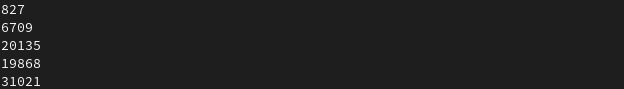Note: Replace 5 with the number of random values of your choice.

Generating random integer when the upper limit is given

Consider that you want to generate a random integer within Y (where Y is not included). Format to achieve this is:

```R=\$((\$RANDOM%Y))
```

To include the upper limit Y, the divisor of the modulo operation will be Y+1. The format is:

```R=\$((\$RANDOM%Y+1))
```

Example:

```echo \$((\$RANDOM%10)) #This will display a random integer between 0 and 9.
echo \$((\$RANDOM%11)) #This will display a random integer between 0 and 10.
```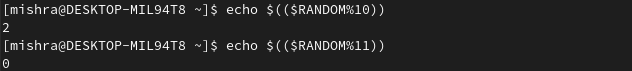When the input is taken from the user in a shell script from the command line to generate the integer, then the following code can be used:

```Y=\$1
div=\$((Y+1))
RANDOM=\$\$
R=\$((\$RANDOM%\$div))
echo \$R
```

Y stores the upper limit and echo \$R displays the random integer. The output is: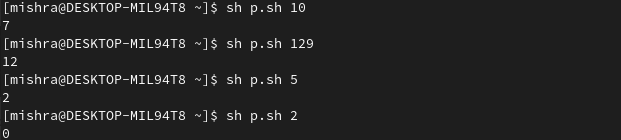Generating random integer when both upper and lower limit is given

Consider that you want to generate a random integer between X and Y where X is the lower limit (X≠0) and Y is the upper limit. Format to achieve this is:

```RANGE=\$((Y-X+1))
R=\$((\$((\$RANDOM%\$RANGE))+X))
```

Example:

```echo "lower limit:"
echo "upper limit:"